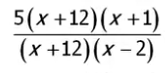Scroll

# When can I/can't I cancel factors with variables such as (x - 1) /(x - 1)?

This is a fantastic question! :)

One of the most important mathematical rules we need to consider when we're trying to cancel out factors is:

• We cannot divide by the number 0, EVER.

Dividing any number by 0 (ex. 6/0, x/0) will result in an undefined answer. This is not mathematically allowed and also a large reason why we aren't able to cancel factors (x-1)/(x-1) even though they're the same.

This can be kind of confusing - I know. But allow me to explain further. :D

So, let's say we have a fraction:And we factor the binomials:At this point, I know it can be tempting to cancel out (x+12)/(x+12) and turn it into 1, but we can't. Why can't we? The reason we can't is because we don't know what x equals yet. What happens when x = -12?

If x = -12, then we would have:

5(0)(x + 1) / 0(x - 2)

0/0 = undefined

As you can see, that would be a problem. Remember, we can't divide ANY number by 0, not even 0. Therefore, if x = -12, we're going to run into problems when we try to cancel because it wouldn't equal 1 - it would be undefined instead! That's why we can't cancel here. However, if the problem had stated that x did not equal -12, then we would be able to cancel.

In other words, we're allowed to cancel numbers, but not variables or variable expressions if there's a chance the denominator will equal 0.

So, say we had a numeric situation where we had 5/5, 6/6, or etc. In this case, there's absolutely no risk that we're dividing by 0. Therefore, we can do the cancellation! :D

But if there's a variable involved in the denominator (the bottom half of the division), then we could run into trouble. For example, if we have something like 3x/x, we CANNOT cancel the x's. What if x=0? Unless we're EXPLICITLY told that x does NOT equal zero (which does occur sometimes on the GRE), we CANNOT assume that it doesn't equal 0. Therefore, it's not possible to cancel x/x in this equation.

The same is true when we add something to a variable, as in this problem. If we have 5(x-2)/(x-2), we can't cancel (x-2)/(x-2), because if x=2, we'll have 0/0, which is not possible mathematically.

Notice, however, that we can cancel something where there's only a variable in the top. For example, 3x/3 would simplify to just x. The bottom could not equal 0, so we're safe - we're cancelling out 3/3. This is distinct from 3x/x, which we could NOT cancel, because there's a possibility that the x on the bottom could equal 0.

The main point is this:

When you are trying to cancel factors, always be on the lookout for a possible combination that could equal zero in the bottom half of your cancellation. If there's any possibility that the quantity on the bottom could equal 0, you CANNOT cancel. In addition, if you aren't explicitly told that the denominator can't equal 0, then you cannot cancel. :D